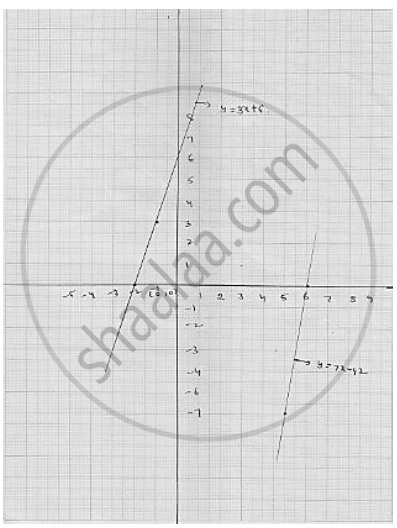# Ravish tells his daughter Aarushi, “Seven years ago, I was seven times as old as you were then. Also, three years from now, I shall be three times as old as you will be”. If present ages of Aarushi - Mathematics

Sum

Ravish tells his daughter Aarushi, “Seven years ago, I was seven times as old as you were then. Also, three years from now, I shall be three times as old as you will be”. If present ages of Aarushi and Ravish are x and y years respectively, represent this situation algebraically as well as graphically.

#### Solution

It is given that seven year ago Ravish was seven times as old as his daughter

∴ 7 ( x - y )  = y - 7

⇒  7x - 49 = y -7

⇒ 7x - 42 = y            .............  (1)

It is also given that after three years from now Ravish shall be three times a sold as her daughter

∴ 3 ( x + 3 ) = y + 3 ⇒ 3x + 9 = y + 3 ⇒ 3x + 6 = y        ........... (2)

Now , y = 7x - 42                 [  using (1) ]

Putting x = 6 , we get y = 7 × 6 - 42 = 0

Putting x = 5, we get y = 7 × 5 - 42 = - 7

Thus, we have following table for the points on the

Line   7x - 42 = y

 x 6 5 y 0 -7

We have,

y = 3x + 6                  [ using (2) ]

Putting  x = - 2 , we get  y = 3  × ( -2 ) + 6 = 0

Putting x = -1 , we get  y = 3× (-1) + 6 = 3

Thus, we have following table for the points on the

Line  y = 3x + 6

 x -1 -2 y 3 0Concept: Linear Equation in Two Variables
Is there an error in this question or solution?

#### APPEARS IN

RD Sharma Mathematics for Class 9
Chapter 7 Linear Equations in Two Variables
Exercise 7.3 | Q 20

Share